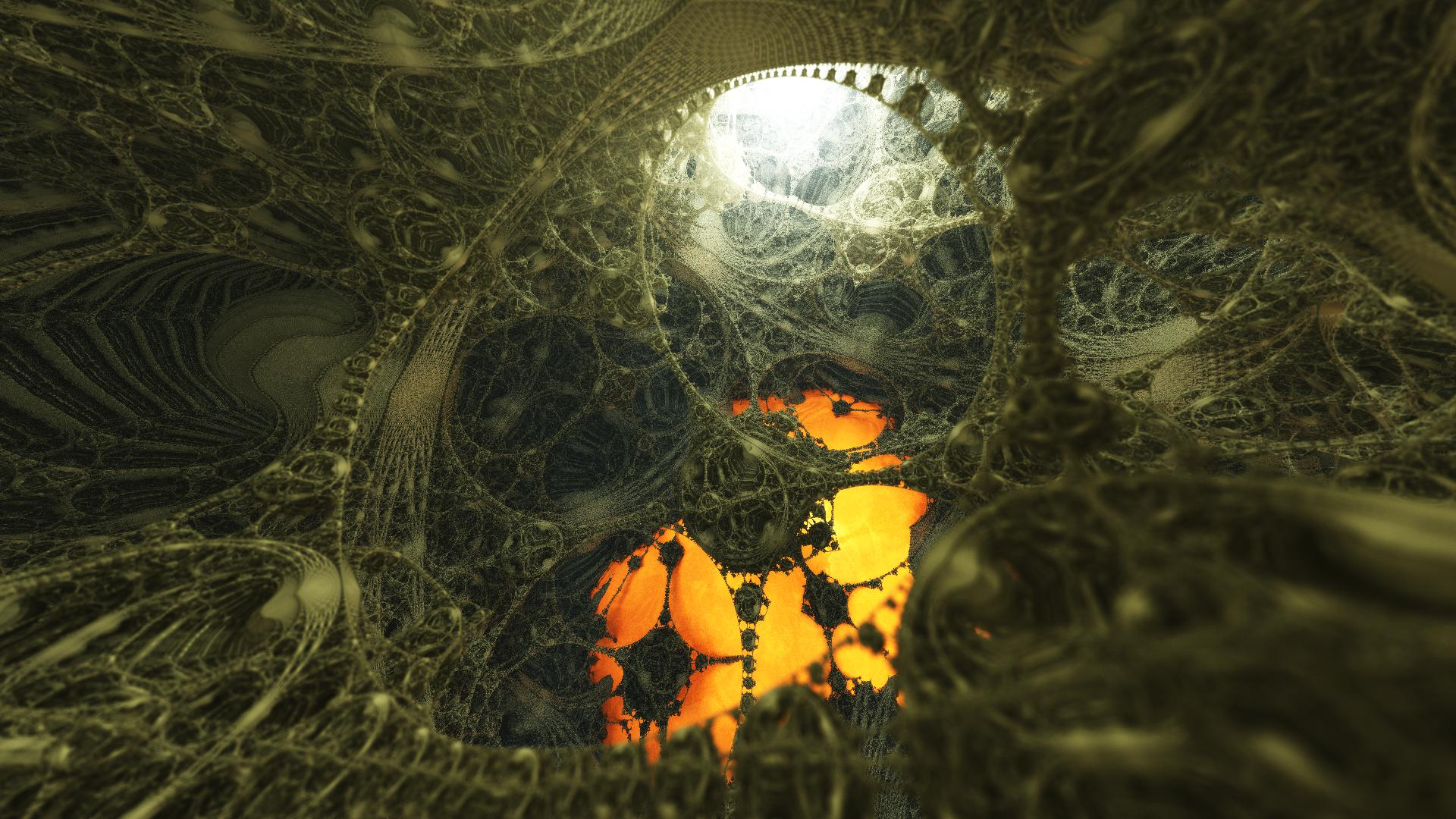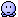News: Visit the official fractalforums.com Youtube Channel## The All New FractalForums is now in Public Beta Testing! Visit FractalForums.org and check it out!

 Pages:    Go DownAuthor Topic: Under there  (Read 470 times) Description: 0 Members and 1 Guest are viewing this topic.
Tim Emit
ConquerorPosts: 111« on: November 09, 2016, 11:32:10 PM »

Under therehttp://www.fractalforums.com/index.php?action=gallery;sa=view;id=19757

Fragmentarium. working on Bulatov's Limit-sets.frag with the de-kn8 raytracer..
getting there but arghh a hard render and what am i doing wrong with it..any positional light increase from 0 on size creates nasty grain. How should i better adjust distance factor/ao etc to get it a cleaner fractal ?
very lovely fragment to play with but it does seem to crash my card and renders slow.
Code:
#version 420
// Created: Sat Nov 5 22:15:00 2016
#define providesInit
#define providesColor
#include "DE-Kn8.frag"
/**
Limit Set of 4D Hyperbolic Reflection Group
December 15, 2012

4D hyperbolic reflection group is generated by reflection in a set of hyperplanes in
4D hyperbolic space (H4). In order to form a group, dihedral angles of hyperplanes intersections
have to be integer fraction of pi (pi/n).

H4 space can be modeled in 4D euclidean space as half space bounded by
3D euclidean hyperplane ("the plane at infinity").
Hyperbolic planes in that model are represented as euclidean 4D hyperspheres
and hyperplanes which orthogonal to the plane at infinity.
This plane at infinity is our usual flat euclidean 3D space. Intersections of 4D hyperplanes
with this plane at infinity are usual 3D euclidean planes and spheres.
We can model the 4D reflection group staying all the time in convenient
3D euclidean space.

"The Limit Set" of reflection group are points of accumulation of action
of the elements of the group at some arbitrary initial point. If we take an initial point
and apply a to it all possible sequence of reflection (infinite set) we
will get a infinite set of points in 3D. Some of the images of the initial
points will be isolated points. But there will be points, which have other points in
arbitrary small neighborhood. Such points can be reached only after applying
an infinite number of reflection.

We are hunting for the set of such points - "The Limit Set".

Here we have example of reflection group which has octahredral symmetry in
our 3D space.

*/

#group limitset
uniform int angle_cube; slider[0,4,100]
uniform int angle_octa; slider[0,4,100]
//size of cube
uniform float size_cube; slider[0.,0.5,20]
// size of octahedron
uniform float size_octa; slider[0.,5.,20]

// radius of cube spheres 1/sqrt(3), which have intersection angle pi/3
uniform float R_cube; slider[0.,0.57735,1]
// radius of octa spheres which have intersection angle pi/3
// 1/sqrt(6)
uniform float R_octa; slider[0.,0.408248,1]
uniform float scale; slider[0.,1,2]
uniform float r_outside; slider[0.,1,20]
uniform int maxCount; slider[0,20,200]
uniform float distanceFactor; slider[0.,1.,10.]

// spheres in vertices of cube
uniform bool g_ppp; checkbox[true]
// spheres in vertices of octahedron
uniform bool g_00p; checkbox[true]
uniform bool g_x; checkbox[true]
uniform bool g_yz; checkbox[true]
uniform bool g_xy; checkbox[true]

uniform bool g_outside; checkbox[false]

uniform float time;

struct SPlane { // sphere or plane
int type;     // 0 - sphere, 1 plane
vec3 c;  // center of sphere or normal vector to plane
float d; // radius of sphere or distance to plane from origin
};

struct FundamentalDomain {
SPlane s;  // sides of fundamental domain
int count;    // count of sides
};

// this is the set of group generators
FundamentalDomain fundDomain;

/**
reflect in the splane
*/
void reflect(SPlane s, inout vec3 v, inout float scale){

if(s.type == 0){ // sphere
v = v - s.c;
float len2 = dot(v,v);
float r2 = s.d;
r2 *= r2;
float factor = (r2/len2);
v *= factor;
scale *= factor;
v += s.c;
} else { // plane
float vn = dot( v - s.c*s.d, s.c);
v -= 2*s.c*vn;
}
}

/**
distance to the splane
*/
float distance(SPlane s, vec3 v){

if(s.type == 0){ // sphere
v -= s.c;
float d = dot(v,v) - s.d*s.d;
if(s.d < 0)
return d;   // outside of sphere
else
return -d;  // inside of sphere

} else { // plane

return dot(v,s.c) - s.d;

}
}

}

SPlane plane(vec3 normal, float distance){

float d = sqrt(dot(normal,normal));
normal *= 1/d; // normalize normal
return SPlane(1,normal, distance);

}

FundamentalDomain getFundamentalDomain() {

float pi = 3.1415926;
float period = 40; // 20 sec
float sc = size_cube; // centers of cube corner spheres are (s,s,s)
float rc = R_cube;

if(angle_cube >= 2){
rc = 2*sc/sqrt(2*(1+cos(pi/angle_cube)));
}
float ro = R_octa;
float so = size_octa;

if(angle_octa >= 2){
ro = so*sqrt(2)/sqrt(2*(1+cos(pi/angle_octa)));
}

so *= scale;
sc *= scale;
ro *= scale;
rc *= scale;

FundamentalDomain fd;
int c = 0;

if(g_ppp)fd.s[c++] = sphere(vec3( sc, sc, sc), -rc);
if(g_00p)fd.s[c++] = sphere(vec3( 0, 0, so), -ro);
if(g_x)fd.s[c++] = plane(vec3( 1, 0,0), 0);
if(g_yz)fd.s[c++] = plane(vec3( 0, -1.,1.), 0);
if(g_xy)fd.s[c++] = plane(vec3( -1,1,0), 0);

if(g_outside)fd.s[c++] = sphere(vec3(0, 0,0), r_outside*scale);

fd.count = c;

return fd;
}

void init() {

fundDomain = getFundamentalDomain();

}

vec3 baseColor(vec3 p, vec3 n) {

return vec3(0.0,0.,1.);

}

float DE_limitSet(vec3 p){

float s = 1.;

int count  = maxCount;

while(count > 0){

int found = 0;

for(int i =0; i < fundDomain.count; i++){
float ip = distance(fundDomain.s[i], p);
if(ip < 0){
reflect(fundDomain.s[i], p, s);
found = 1;
}
}

if(found == 0){
// no new reflections found - we are in fundamental domain
break;
}

count--;
}

// one more reflection to properly normalize points at infinity
s *= 2./(1.+dot(p,p));

float dist = 1/s;

dist *= distanceFactor;

return dist;

}

float DE(vec3 p) {
return DE_limitSet(p);
}

#preset timemit
FOV = 1.558424
Eye = -0.1042755,0.3768983,0.160479
Target = 1.255068,-2.191981,-0.3550798
Up = 0.501557,0.0646907,1
EquiRectangular = false
Gamma = 1.042966
ToneMapping = 5
Exposure = 0.4773203
Brightness = 0.9033613
Contrast = 1.869777
Saturation = 0.5672269
GaussianWeight = 1.6949
AntiAliasScale = 0.1351351
Detail = -3.70076
DetailAO = -1.192737
FudgeFactor = 0.0868031
Dither = 0.4561767
NormalBackStep = 0.1290323 NotLocked
AO = 0,0,0,1
CamLight = 1,0.9803922,0.9411765,1.814972
CamLightMin = 0.3365922
Glow = 1,1,1,0.43836
GlowMax = 31
BaseColor = 0.3019608,0.3098039,0.2078431
OrbitStrength = 0.0525947
X = 1,1,1,1
Y = 0.345098,0.666667,0,0.02912
Z = 1,0.666667,0,1
R = 0.0784314,1,0.941176,-0.0194
BackgroundColor = 0,0,0
CycleColors = false
Cycles = 4.04901
EnableFloor = true NotLocked
FloorNormal = -0.0160727,-0.0859539,-0.0607966
FloorHeight = 0.2965806
FloorColor = 0.9568627,0.454902,0.1176471
AutoFocus = false
FocalPlane = 1.733793
Aperture = 0.0027875
InFocusAWidth = 0.7081851
ApertureNbrSides = 5
ApertureRot = 0
ApStarShaped = false
Bloom = true
BloomIntensity = 0.2871357
BloomPow = 0.7303371
BloomTaps = 8
MaxRaySteps = 1779
MaxDistance = 20
AoCorrect = 0.4913375
Specular = 0
SpecularExp = 16
Reflection = 0.5686275,0.4941176,0.3372549
ReflectionsNumber = 2
SpotGlow = true
SpotLight = 0.4823529,0.5254902,0.5843137,2.001419
LightPos = 0.0832178,0.1664355,0.4022191
LightSize = 0
LightFallOff = 0.3048017
LightGlowExp = 0.4306723
HF_Fallof = 0.21817
HF_Const = 0.2062284
HF_Intensity = 0.3293706
HF_Dir = 0,0,1
HF_Offset = 0
HF_Color = 0.8313725,0.9333333,1,0.9067797
HF_Scatter = 0
HF_Anisotropy = 0,0,0
HF_FogIter = 1
CloudScale = 1
CloudFlatness = 0
CloudTops = 1
CloudBase = -1
CloudDensity = 1
CloudRoughness = 1
CloudContrast = 1
CloudColor = 0.65,0.68,0.7
SunLightColor = 0.7,0.5,0.3
angle_cube = 13
angle_octa = 10
size_cube = 1.094737
size_octa = 1.513665
R_cube = 0.2302358
R_octa = 0.3608033
scale = 0.0729023
r_outside = 2.887176
maxCount = 151
distanceFactor = 0.2855103
g_ppp = true
g_00p = true
g_x = true
g_yz = true
g_xy = true
g_outside = true
#endpresetLogged
3dickulus
Global Moderator
Fractal SeniorPosts: 1558« Reply #1 on: November 10, 2016, 05:31:47 AM »

very nicewhen compiling this frag I see lots of warnings, these should be fixed before further testing...
also 2 reflections and very low fudge factor makes sloooow render (but you probably need these to get the look you want)

using #version 120 gets rid of about 12 warnings
fixing "implicit cast int to float" gets rid of the rest

I don't have DE-kn8 only DE-Kn8-test so not sure if it's the same as yours

try the attached zip, I made some adjustments doesn't look as good thoughLimit_Sets_Files.zip (139.47 KB - downloaded 54 times.)Logged

Resistance is fertile...
You will be illuminated!

#B^] https://en.wikibooks.org/wiki/Fractals/fragmentarium
Sabine
Fractal FertilizerPosts: 373« Reply #2 on: November 10, 2016, 10:01:40 AM »Beautiful place you found there! Must look at your .fragLogged

sabine62.deviantart.com
 Pages:    Go Down